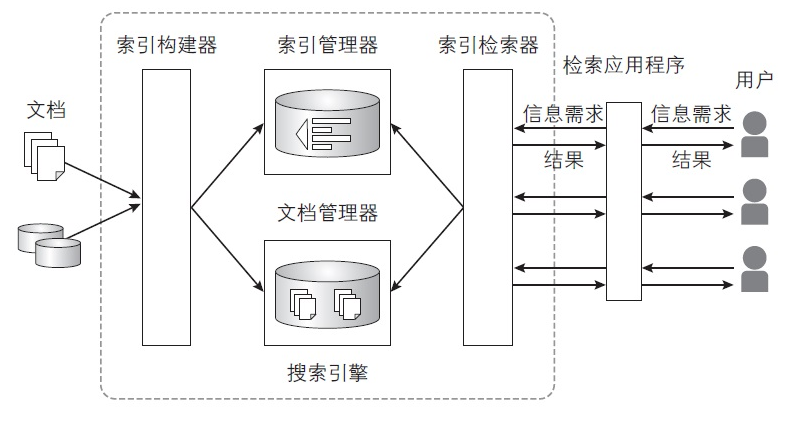# Go 实现的数学表达式解析计算引擎math-engine国

Apache

2019-07-08

math-engine 正在参加 2019 年度最受欢迎开源中国软件评选，请投票支持！
math-engine 在 2019 年度最受欢迎开源中国软件评选 中已获得 {{ projectVoteCount }} 票，请投票支持！

【年终提升】2019尾声，来 OSC·年终盛典收割技术干货，get新技能！>>>• `1+127-21+(3-4)*6/2.5`
• `(88+(1+8)*6)/2+99`
• `123_345_456 * 1.5 - 2 ^ 4`
• `-4 * 6 + 2e2 - 1.6e-3`
• `sin(pi/2)+cos(45-45*1)+tan(pi/4)`
• `99+abs(-1)-ceil(88.8)+floor(88.8)`
• `max(min(2^3, 3^2), 10*1.5-7)`

### Demo## Method Support

symbol explanation e.g.
`+` 加，plus 1+2 = 3
`-` 减，sub 8-3.5 = 4.5
`*` 乘，multiply 2*3 = 6
`/` 除，division 5/2 = 2.5
`%` 取余，remainder 5%2 = 1
`^` 整数次方，integer power 2^3 = 8, 3^2 = 9
`e` 科学计数法，E-notation 1.2e3 = 1200，1.2e-2 = 0.012
`()` 括号，brackets (2+3)*4 = 20
`_` 数字分隔符，number separator 123_456_789 = 123456789
`pi` π pi = 3.141592653589793
`sin(x)` 正弦函数，sine sin(pi/2) = 1
`cos(x)` 余弦函数，cosine cos(0) = 1
`tan(x)` 正切函数，tangent tan(pi/4) = 1
`cot(x)` 余切函数，cotangent cot(pi/4) = 1
`sec(x)` 正割函数，secant sec(0) = 1
`csc(x)` 余割函数，cosecant csc(pi/2) = 1
`abs(x)` 绝对值，absolute value abs(-6) = 6
`ceil(x)` 向上取整 ceil(4.2) = 5
`floor(x)` 向下取整 floor(4.8) = 4
`round(x)` 四舍五入取整 round(4.4) = 4, round(4.5) = 5
`sqrt(x)` 平方根，square root sqrt(4) = 2
`cbrt(x)` 立方根，cube root cbrt(27) = 3
`max(x, y)` x, y 中的较大值 max(2, 3) = 3
`min(x, y)` x, y 中的较小值 min(2, 3) = 2

## Usage

`go get -u github.com/dengsgo/math-engine`

`import "github.com/dengsgo/math-engine/engine"`

e.g. 1 直接调用解析执行函数 :

```import "github.com/dengsgo/math-engine/engine"

func main() {
s := "1 + 2 * 6 / 4 + (456 - 8 * 9.2) - (2 + 4 ^ 5)"
// call top level function
r, err := engine.ParseAndExec(s)
if err != nil {
fmt.Println(err)
}
fmt.Printf("%s = %v", s, r)
}```

e.g. 2 依次调用函数，手动执行 :

```import "github.com/dengsgo/math-engine/engine"

func main() {
s := "1 + 2 * 6 / 4 + (456 - 8 * 9.2) - (2 + 4 ^ 5)"
exec(s)
}

// call engine
// one by one
func exec(exp string) {
// input text -> []token
toks, err := engine.Parse(exp)
if err != nil {
fmt.Println("ERROR: " + err.Error())
return
}
// []token -> AST Tree
ast := engine.NewAST(toks, exp)
if ast.Err != nil {
fmt.Println("ERROR: " + ast.Err.Error())
return
}
// AST builder
ar := ast.ParseExpression()
if ast.Err != nil {
fmt.Println("ERROR: " + ast.Err.Error())
return
}
fmt.Printf("ExprAST: %+v\n", ar)
// AST traversal -> result
r := engine.ExprASTResult(ar)
fmt.Println("progressing ...\t", r)
fmt.Printf("%s = %v\n", exp, r)
}```

```ExprAST: {Op:- Lhs:{Op:+ Lhs:{Op:+ Lhs:{Val:1} Rhs:{Op:/ Lhs:{Op:* Lhs:{Val:2} Rhs:{Val:6}} Rhs:{Val:4}}} Rhs:{Op:- Lhs:{Val:456} Rhs:{Op:* Lhs:{Val:8} Rhs:{Val:9.2}}}} Rhs:{Op:+ Lhs:{Val:2} Rhs:{Op:^ Lhs:{Val:4} Rhs:{Val:5}}}}
progressing ...  -639.6
1+2*6/4+(456-8*9.2)-(2+4^5) = -639.6```

## TrigonometricMode

```import "github.com/dengsgo/math-engine/engine"

func main() {
s := "1 + sin(90)"
engine.TrigonometricMode = engine.AngleMode
engine.ParseAndExec(s) // will return 2, nil
s = "1 + sin(pi/2)"
engine.ParseAndExec(s) // will return 2, nil
}```

## Document

godoc.org/github.com/dengsgo/math-engine/engine

## Compile

go version 1.12

```# Compile Demo
go test
go build
./math-engine```

Github Releases

## TODO

### 已实现

•  加 `+`
•  减 `-`
•  乘 `*`
•  除 `/`
•  取余 `%`
•  整数次方 `^`
•  科学计数法 e.g. `1.2e7`、 `1.2e-7`
•  括号 `()`
•  混合运算 e.g. `1+2*6/4+(456-8*9.2)-(2+4^5)*2e3+1.2e-2`
•  友好的长数字 e.g. `123_456_789`
•  三角函数 e.g. `sin, cos, tan, cot, sec, csc`
•  常量 pi
•  辅助函数 e.g. `abs, ceil, floor, sqrt, cbrt`
•  友好的错误消息 e.g.
```input /> 123+89-0.0.9
ERROR: strconv.ParseFloat: parsing "0.0.9": invalid syntax
want '(' or '0-9' but get '0.0.9'
------------
123+89-0.0.9
^
------------```

•  精确浮点计算
•  更多辅助函数

### 评论(0)### math-engine v2.0，数学表达式解析计算引擎

07/15 09:38#### 暂无问答

2012/03/28 10:23
594
0
MAX函数和GROUP BY 语句一起使用的一个误区

2014/04/05 23:58
130
0

2016/01/11 16:50
225
1MYSQL_使用外键约束(constraint)或触发器(trigger)来进行级联更新、删除

2015/06/08 10:07
1K
1
game engine architecture---1.6

2012/03/09 14:07
99
0

Game Developer Magazine 1994 - 2000年，共7年的游戏开发者杂志电子版（含源码） Graphics Programming Black Book (by Michael Abrash)，图形编程黑书，Id software的Michael Abrash编著 ...

2016/06/20 13:40
13
0

Game Developer Magazine 1994 - 2000年，共7年的游戏开发者杂志电子版（含源码） Graphics Programming Black Book (by Michael Abrash)，图形编程黑书，Id software的Michael Abrash编著 ...

2016/06/20 13:39
46
0
Aspose.Words for Java v19.9新功能：实现创建重复节结构化文档标签能力！

Aspose.Words for Java是功能丰富的Word处理API，允许开发人员在不使用Microsoft Word的情况下嵌入在自己的Java应用程序中生成，修改，转换，呈现和打印文档的功能。同时还提供访问和操作所有...

09/16 15:23
24
0

Game Developer Magazine 1994 - 2000年，共7年的游戏开发者杂志电子版（含源码） Graphics Programming Black Book (by Michael Abrash)，图形编程黑书，Id software的Michael Abrash编著 ...

2016/06/20 13:39
6
0